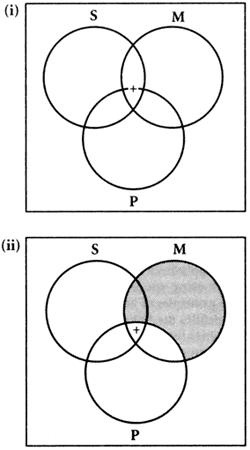# Venn diagram

﻿
Venn diagram
A kind of diagram invented by Venn in 1881, for representing and assessing the validity of elementary inferences either of a syllogistic form, or from the Boolean algebra of classes (Venn was attempting to illustrate Boole's own methods). In a Venn diagram for the syllogism there are three circles, corresponding to S, M, and P. Shaded areas indicate which combinations are empty, and a cross indicates which ones have members, while a cross on a border between two classes represents that at least one class has a member. For example, to illustrate the syllogism ‘Some S is M, all M is P, so some S is P’, the first premise is represented by (i). Here the cross hovers between the area of P and the area outside P. However, the second premise adds the shading of (ii), since any M region outside P is empty. Hence, the cross cannot be there, and is driven into the P area. This shows that some S is indeed P; hence the syllogism is valid. Venn generalized the method. For statements in the algebra of classes involving four terms, ellipses can be drawn, but the method becomes cumbersome. Strips and charts of various kinds were invented in the late 19th and early 20th centuries to give graphical representations of such problems.Philosophy dictionary. . 2011.

### Look at other dictionaries:

• Venn diagram — Venn diagrams or set diagrams are diagrams that show all hypothetically possible logical relations between a finite collection of sets (groups of things). Venn diagrams were invented around 1880 by John Venn. They are used in many fields,… …   Wikipedia

• Venn diagram — Venn o diagrama statusas T sritis automatika atitikmenys: angl. Venn diagram vok. Venn Diagramm, n rus. диаграмма Венна, f pranc. diagramme de Venn, m ryšiai: sinonimas – Veno diagrama …   Automatikos terminų žodynas

• Venn diagram — ► NOUN ▪ a diagram representing mathematical or logical sets as circles, common elements of the sets being represented by intersections of the circles. ORIGIN named after the English logician John Venn (1834 1923) …   English terms dictionary

• Venn diagram — [ven] n. [after John Venn (1834 1923), Eng logician] Math. a diagram, esp. in symbolic logic, using overlapping circles, often shaded or crosshatched, to show relationships between sets …   English World dictionary

• Venn diagram — 1884, named for English logician John Venn (1834 1923) …   Etymology dictionary

• Venn diagram — UK [ˈven ˌdaɪəɡræm] / US noun [countable] Word forms Venn diagram : singular Venn diagram plural Venn diagrams maths a drawing of circles that cross over each other, showing features shared by different sets (= groups of numbers) …   English dictionary

• Venn Diagram — An illustration that uses overlapping or non overlapping circles to show the relationship between finite groups of things. This type of diagram gets its name from John Venn, who introduced the illustration in 1880. Where the circles overlap,… …   Investment dictionary

• Venn diagram — Venn′ di agram [[t]vɛn[/t]] n. math. pho math., logic a diagram that uses circles to represent sets and their relationships • Etymology: 1940–45; after John Venn (1834–1923), English logician …   From formal English to slang

• Venn diagram — Venn di|a|gram [ˈven ˌdaıəgræm] n [Date: 1900 2000; Origin: John Venn (1834 1923), British logician] a picture showing the relationship between several things by using circles that partly cover each other …   Dictionary of contemporary English

• Venn diagram — Venn di|a|gram [ ven ,daıəgræm ] noun count a drawing of circles that cross over each other, showing features shared by different SETS (=groups of numbers or information) …   Usage of the words and phrases in modern English

We are using cookies for the best presentation of our site. Continuing to use this site, you agree with this.